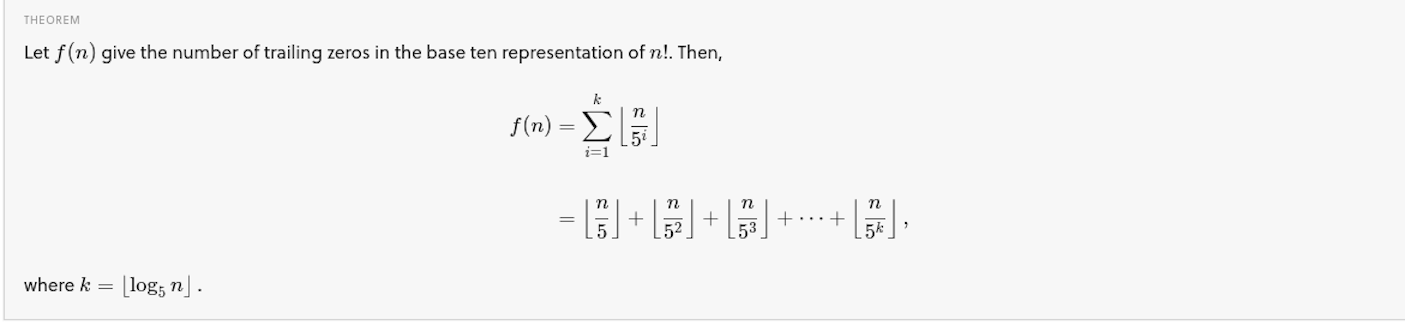# Number of trailing zeros in the factorial of an integer

I recently learned about a cool formula to calculate the number of trailing zeros in the factorial of a number. It has been a while since I wrote a program to do something like this. So, I decided to change that and write this blog post. Let's jump in.

In the spirit of wring various "calculators" in the book, we will write a "number of trailing zero" calculator. First up though, let's refresh some key relevant concepts.

Factorial: The factorial of a number, n denoted by n! is the product n*(n-1)*(n-2)...*1. For example, 5! = 5*4*3*2*1 = 120.

Trailing zeros: The trailing zeros of a number is the number of zeros at the end of a number. For example, the number 567100 has two trailing zeros.

Floor: The floor of a number is the greatest integer less than or equal to x. That is floor of 3.2 is 3 and that of 3.5 is 3 and the floor of 3 is 3 as well.

Now, coming back to the focus of this post, this document at brilliant.org wiki explains the process in detail.

The key bit there in is this formula:where, n is the number for whose factorial we want to find the number of trailing zeros.

The following Python program implements the above formula:

```import math

def is_positive_integer(x):
try:
x = float(x)
except ValueError:
return False
else:
if x.is_integer() and x > 0:
return True
else:
return False

def trailing_zeros(num):
if is_positive_integer(num):
# The above function call has done all the sanity checks for us
# so we can just convert this into an integer here
num = int(num)

k = math.floor(math.log(num, 5))
zeros = 0
for i in range(1, k + 1):
zeros = zeros + math.floor(num/math.pow(5, i))
return zeros
else:
print("Factorial of a non-positive non-integer is undefined")

if __name__ == "__main__":
fact_num = input(
"Enter the number whose factorial's trailing zeros you want to find: "
)
num_zeros = trailing_zeros(fact_num)
print("Number of trailing zeros: {0}".format(num_zeros))
```

When we run this program using Python 3, it will ask for the number whose factorial's number of trailing zeros we want to find and then print it out, like so:

```Enter the number whose factorial's trailing zeros you want to find: 5
Number of trailing zeros: 1
```

If you enter a number which is not a positive integer, you will get an error message:

```Enter the number whose factorial's trailing zeros you want to find: 5.1
Factorial of a non-positive integer is undefined
Number of trailing zeros: None
```

Some key standard library functions we use in the above program are:

• math.floor: This function is used to find the floor of a number
• math.log: This function is used to find the logarithm of a number for a specified base (defaults to 10)
• math.pow: This function is used to find out the power of a number raised to another

The above functions are defined in the math module.

Besides the above, we use the is_integer() function defined on a floating point object to check if the floating point object is actually an integer.